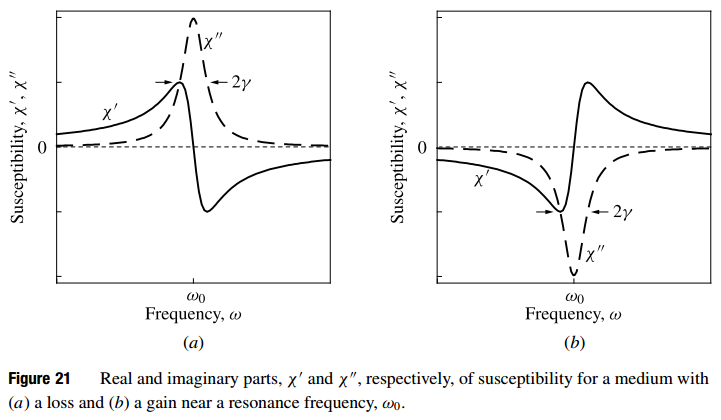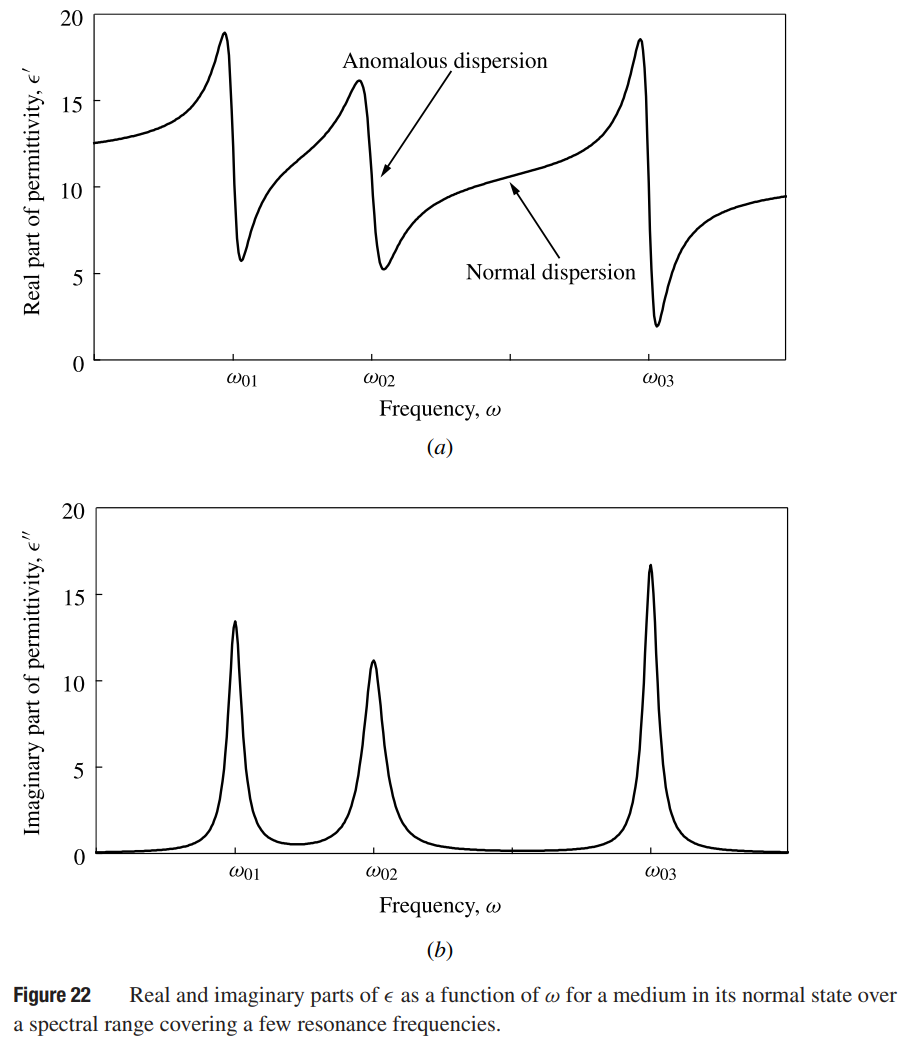# Material Dispersion

This is a continuation from the previous tutorial -

As discussed in the tutorial of optical fields and Maxwell's equations, and linear optical susceptibility, dispersion in the susceptibility of a medium is caused by the fact that the response of the medium to excitation by an optical field does not decay instantaneously.

The general characteristics of the medium can be understood from its impulse response. In general, the impulse response of a medium decays exponentially while oscillating at some resonance frequencies. There may exist several exponential relaxation constants and several oscillation frequencies for a given material across the electromagnetic spectrum. This is true even within the optical spectral region.

However, at a given optical frequency ω, the characteristics of the material response are dominated by the resonance frequency closest to ω and the relaxation constant associated with the oscillation at this particular resonance frequency. We therefore consider, for simplicity, a medium of a single resonance frequency at ω0 with a relaxation constant γ.

The susceptibility in the time domain is simply the impulse response of the medium, which is real and has the following general form:

\tag{174}\chi(t)\propto\left\lbrace\begin{align}&e^{-\gamma{t}}\sin{\omega_0{t}},{\qquad}t>0\\&0,{\qquad\qquad\qquad}t<0\end{align}\right.

Note that χ(t) = 0 for t < 0 because a medium can respond only after, but not before, an excitation. This is the causality condition, which applies to all physical systems.

The Fourier transform of (174) yields

$\tag{175}\chi(\omega)=\displaystyle\int\limits_{-\infty}^{\infty}\chi(t)e^{\text{i}\omega{t}}\text{d}t\approx-\chi_b\frac{\omega_0}{\omega-\omega_0+\text{i}\gamma}$

in the frequency domain, where χb = χ(ω ≪ ω0) is a constant equal to the background value of χ(ω) at low frequencies far away from resonance.

In (175), we have taken the so-called rotating-wave approximation by dropping a term that contains ω + ω0 in its denominator because ω + ω0 ≫ |ω - ω0| in the optical spectral region. This susceptibility has the following real and imaginary parts:

$\tag{176}\chi'(\omega)=-\chi_b\frac{\omega_0(\omega-\omega_0)}{(\omega-\omega_0)^2+\gamma^2},{\qquad}\chi''(\omega)=\chi_b\frac{\omega_0\gamma}{(\omega-\omega_0)^2+\gamma^2}$

which are plotted in figure 21 below.Note that χ''(ω) has a Lorentzian lineshape, which has a FWHM Δω = 2γ. The sign of χ'' depends on that of χb. In the normal state, χb > 0, and the medium has an optical loss near resonance. This characteristic results in the absorption of light at frequency ω = ω0. When χb < 0, the medium has optical gain, resulting in the amplification of light at ω = ω0 such as in the case of laser.

Note that both χ' and χ'' are proportional to χb. Therefore, when χ'' changes sign, χ' also changes sign. When χ'' < 0, χ' is negative for ω < ω0 and positive for ω > ω0, as is shown in figure 21(b).

Example

For an atomic transition associated with absorption or emission of optical radiation at 1 µm wavelength, the resonance frequency is ν0 = c/λ = 300 THz, thus ω0 = 2πν0 = 1.885 x 1015 s-1. If the polarization associated with this resonant transition relaxes with a time constant of τ = 1 ps, then γ = 1/τ = 1012 s-1 and Δω = 2γ = 2 x 1012 s-1. Thus the Lorentzian spectral line has a FWHM linewidth of Δν = Δω/2π ≈ 318 GHz, which is considered quite broad but is approximately only 0.1% of the center frequency ν0 of the spectral line. If the relaxation time constant is τ = 1 ns, we find a spectral linewidth of Δν ≈ 318 MHz. For a relaxation time constant of τ = 1 µs, we have a narrow linewidth of Δν ≈ 318 kHz.

Note that the spectral linewidth is determined by the polarization relaxation time rather than by the population relaxation time of a material. The polarization relaxation time constant is generally much smaller than the population relaxation time constant for a given transition. Therefore, the spectral linewidth of a given transition can be quite broad even when the energy levels involved have long population relaxation times. One good example is the optical transitions in Nd: YAG.

A medium generally has many resonance frequencies, each corresponding to an absorption frequency in the normal state of the medium. Because ε(ω) = ε0(1 + χ(ω)), the dispersion characteristics of ε(ω) depend directly on those of χ(ω) given by (176). Its real and imaginary parts in the normal state as a function of ω over a spectral range covering a few resonances are shown in figure 22 below.Some important dispersion characteristics of χ(ω) and ε(ω) are summarized below.

1. It can be seen from figure 21(a) that χ'(ω ≪ ω0) is larger than χ'(ω ≫ ω0) in the normal state. Therefore, around any single resonance frequency, ε' at any frequency on the low-frequency side has a value larger than that at any frequency on the high-frequency side.

2. A  medium is said to have normal dispersion in a spectral region where ε' increases with frequency so that dε'/dω > 0. It is said to have anomalous dispersion in a spectral region where ε' decreases with increasing frequency so that dε'/dω < 0. Because dn/dω and dε'/dω have the same sign, the index of refraction also increases with frequency in a spectral region of normal dispersion and decreases with frequency in a spectral region of anomalous dispersion.

3. It can be seen from figure 22 that when a material is in its normal state, normal dispersion appears everywhere except in the immediate neighborhood within the FWHM of a resonance frequency where anomalous dispersion occurs. This characteristic can be reversed near a resonance frequency where resonant amplification, rather than absorption, exists.

4. Note the distinction between the definition of normal and anomalous dispersion in terms of the sign of dε'/dω or dn/dω and that of positive and negative group-velocity dispersion in terms of the sign of D. [refer to the phase-velocity, group-velocity and dispersion tutorial]. Both positive and negative group-velocity dispersion can appear in a spectral region where the dispersion defined in terms of dn/dω is normal. In some literature, positive group-velocity dispersion is sometimes referred to as normal dispersion while negative group-velocity dispersion is referred to as anomalous dispersion. This is confusing and is, strictly speaking, not correct.

5. In most transparent materials, such as glass and water, normal dispersion appears in the visible spectral region and may extend to the near infrared and near ultraviolet regions.

Kramers-Kronig Relations

It can be seen from the discussions above that the real and imaginary parts of χ(ω), or those of ε(ω), are not independent of each other. The susceptibility of any physical system has to satisfy the causality requirement in the time domain. This requirement leads to a general relationship between χ' and χ'' in the frequency domain:

$\tag{177}\chi'(\omega)=\frac{2}{\pi}\mathrm{P}\displaystyle\int\limits_{0}^{\infty}\frac{\omega'\chi''(\omega')}{\omega'^2-\omega^2}\text{d}\omega',{\qquad}\chi''(\omega)=-\frac{2}{\pi}\mathrm{P}\int\limits_{0}^{\infty}\frac{\omega\chi'(\omega')}{\omega'^2-\omega^2}\text{d}\omega'$

where the principal values are taken for the integrals. These relations are known as the Kramers-Kronig relations.

Therefore, once the real part of χ(ω) is known over the entire spectrum, its imaginary part can be found, and vice versa.

Note that the relations in (177) are consistent with the fact that χ'(ω) is an even function, while χ''(ω) is an odd function, of ω, as discussed in the linear optical susceptibility tutorial.

The contradiction to this statement seen in (176) is only apparent but not real. It is caused by the rotating-wave approximation taken in (175). There is no contradiction when the approximation is removed and exact expressions are used for χ'(ω) and χ''(ω).

Photon Nature of Light

When considering the function of a device that involves the emission and absorption of light, a purely electromagnetic wave description of light is not adequate. In this situation, the photon nature of light cannot be ignored. Meanwhile, the material involved in this process also undergoes quantum mechanical transitions between its energy levels.

The energy of a photon is determined by its frequency ν or, equivalently, its angular frequency ω. Associated with the particle nature of a photon, there is a momentum determined by its wavelength λ or, equivalently, its wavevector k. These characteristics are summarized below for a photon in free space:

speed            c = λν

energy           hν = ℏω = pc

momentum    p = hν/c = h/λ         pk

The energy of a photon that has a wavelength λ in free space can be calculated using the following formula:

$\tag{178}h\nu=\frac{1.2398}{\lambda}\mu{m}\,eV=\frac{1239.8}{\lambda}nm\,eV$

For example, at an optical wavelength of 1 µm, the photon energy is 1.2398 eV.

The energy of a photon is determined only by the frequency, or wavelength, of light, but not by its intensity. The intensity of light is related to the flux density, or number per unit time per unit area, of photons by

$\tag{179}\text{photon flux density} = \frac{I}{h\nu}=\frac{I}{\hbar\omega}$

Example

It is found that a piece of crystal transmits light at λ = 500 nm but absorbs light at λ = 400 nm. Make an intelligent guess of its bandgap from this limited information.

Because a crystal transmits photons with energies below its bandgap but absorbs those with energies above its bandgap, we can reasonably guess that the bandgap of this crystal falls between the photon energies corresponding to 500 and 400 nm wavelengths. Using (178) for the photon energy, we find that

2.48 eV < Eg < 3.10 eV.

The next part continues with the Waveguide Modes tutorial.